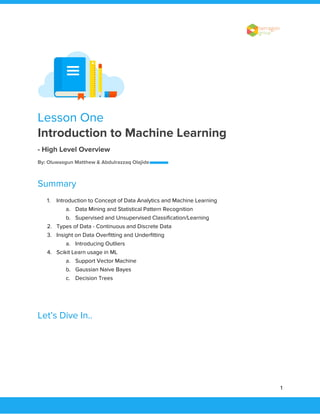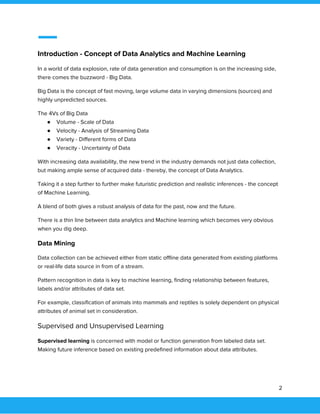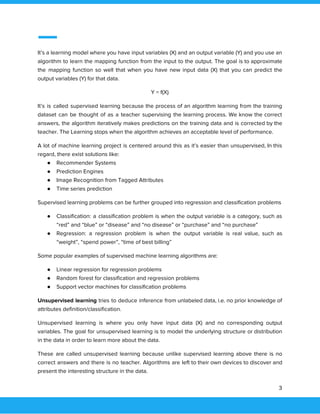Se está descargando tu SlideShare. ×

# Introduction to machine learning

Anuncio
Anuncio
Anuncio
Anuncio
Anuncio
Anuncio
Anuncio
Anuncio
Anuncio
Anuncio
AnuncioAnuncio
Anuncio
Cargando en…3
×

1 de 10 Anuncio

# Introduction to machine learning

In a world of data explosion, the rate of data generation and consumption is on the increasing side,
there comes the buzzword - Big Data.

Big Data is the concept of fast-moving, large-volume data in varying dimensions (sources) and
highly unpredicted sources.

The 4Vs of Big Data
● Volume - Scale of Data
● Velocity - Analysis of Streaming Data
● Variety - Different forms of Data
● Veracity - Uncertainty of Data

With increasing data availability, the new trend in the industry demands not just data collection but making an ample sense of acquired data - thereby, the concept of Data Analytics.
Taking it a step further to further make futuristic prediction and realistic inferences - the concept
of Machine Learning.

A blend of both gives a robust analysis of data for the past, now and the future.
There is a thin line between data analytics and Machine learning which becomes very obvious
when you dig deep.

In a world of data explosion, the rate of data generation and consumption is on the increasing side,
there comes the buzzword - Big Data.

Big Data is the concept of fast-moving, large-volume data in varying dimensions (sources) and
highly unpredicted sources.

The 4Vs of Big Data
● Volume - Scale of Data
● Velocity - Analysis of Streaming Data
● Variety - Different forms of Data
● Veracity - Uncertainty of Data

With increasing data availability, the new trend in the industry demands not just data collection but making an ample sense of acquired data - thereby, the concept of Data Analytics.
Taking it a step further to further make futuristic prediction and realistic inferences - the concept
of Machine Learning.

A blend of both gives a robust analysis of data for the past, now and the future.
There is a thin line between data analytics and Machine learning which becomes very obvious
when you dig deep.

Anuncio
Anuncio

### Introduction to machine learning

1. 1.     Lesson One  Introduction to Machine Learning   - High Level Overview   By: Oluwasgun Matthew & Abdulrazzaq Olajide   Summary  1. Introduction to Concept of Data Analytics and Machine Learning  a. Data Mining and Statistical Pattern Recognition  b. Supervised and Unsupervised Classification/Learning  2. Types of Data - Continuous and Discrete Data  3. Insight on Data Overfitting and Underfitting  a. Introducing Outliers  4. Scikit Learn usage in ML  a. Support Vector Machine  b. Gaussian Naive Bayes  c. Decision Trees    Let’s Dive In..        1
2. 2.     Introduction - Concept of Data Analytics and Machine Learning  In a world of data explosion, rate of data generation and consumption is on the increasing side,  there comes the buzzword - Big Data.  Big Data is the concept of fast moving, large volume data in varying dimensions (sources) and  highly unpredicted sources.  The 4Vs of Big Data  ● Volume - Scale of Data  ● Velocity - Analysis of Streaming Data  ● Variety - Different forms of Data  ● Veracity - Uncertainty of Data  With increasing data availability, the new trend in the industry demands not just data collection,  but making ample sense of acquired data - thereby, the concept of Data Analytics.   Taking it a step further to further make futuristic prediction and realistic inferences - the concept  of Machine Learning.   A blend of both gives a robust analysis of data for the past, now and the future.  There is a thin line between data analytics and Machine learning which becomes very obvious  when you dig deep.  Data Mining  Data collection can be achieved either from static offline data generated from existing platforms  or real-life data source in from of a stream.  Pattern recognition in data is key to machine learning, finding relationship between features,  labels and/or attributes of data set.  For example, classification of animals into mammals and reptiles is solely dependent on physical  attributes of animal set in consideration.  Supervised and Unsupervised Learning  Supervised learning ​is concerned with model or function generation from labeled data set.  Making future inference based on existing predefined information about data attributes.  2
3. 3.     It’s a learning model where you have input variables (X) and an output variable (Y) and you use an                                      algorithm to learn the mapping function from the input to the output. The goal is to approximate                                  the mapping function so well that when you have new input data (X) that you can predict the                                    output variables (Y) for that data.  Y = f(X)  It’s is called supervised learning because the process of an algorithm learning from the training                              dataset can be thought of as a teacher supervising the learning process. We know the correct                                answers, the algorithm iteratively makes predictions on the training data and is corrected by the                              teacher. The Learning stops when the algorithm achieves an acceptable level of performance.  A lot of machine learning project is centered around this as it’s easier than unsupervised, In this                                  regard, there exist solutions like:  ● Recommender Systems  ● Prediction Engines  ● Image Recognition from Tagged Attributes  ● Time series prediction  Supervised learning problems can be further grouped into regression and classification problems  ● Classification: a classification problem is when the output variable is a category, such as                            “red” and “blue” or “disease” and “no disease” or “purchase” and “no purchase”  ● Regression: a regression problem is when the output variable is real value, such as                            “weight”, “spend power”, “time of best billing”  Some popular examples of supervised machine learning algorithms are:  ● Linear regression for regression problems  ● Random forest for classification and regression problems  ● Support vector machines for classification problems  Unsupervised learning tries to deduce inference from unlabeled data, i.e. no prior knowledge of                            attributes definition/classification.   Unsupervised learning is where you only have input data (X) and no corresponding output                            variables. The goal for unsupervised learning is to model the underlying structure or distribution                            in the data in order to learn more about the data.  These are called unsupervised learning because unlike supervised learning above there is no                          correct answers and there is no teacher. Algorithms are left to their own devices to discover and                                  present the interesting structure in the data.  3
4. 4.     The following solutions are classified under this category:  ● Fraud Detection from weird transaction  ● Clustering students into types based on learning styles  Unsupervised learning problems can be further grouped into clustering and association  problems.  ● Clustering: A clustering problem is where you want to discover the inherent groupings in                            the data, such as grouping customers by purchasing behavior  ● Association: An association run learning problem is where you want to discover rules that                            describe large portions of your data, such as people that buy X also tend to buy Y.  Some popular examples of unsupervised learning algorithms are:  ● K-means for clustering problems  ● Apriori algorithm for association rule learning problems.  Quiz ​Classify the following as either supervised or unsupervised learning:  ● Spam detection in emails  ● Fraud detection in transactions  ● Customer segmentation  ● Speech recognition  ● Weather forecast  ● House price prediction  ● Astronomy prediction    Types of Data - Continuous and Discrete Data  There exist a wide range of data format that will be encountered during data collection, and  sanitization from numerical, categorical, time series and text base data.  Quiz ​What type of data type is:  ● CPE508 Result  ● List of courses offered in 500Level - Computer Science and Engineering  ● Gender  ● Frequency of Strike actions in O.A.U  ● Lectures time table  4
5. 5.     Data Overfitting and Underfitting  In machine learning we describe the learning of the target function from training data as inductive                                learning. Induction refers to learning general concepts from specific examples which is exactly                          the problem that supervised machine learning problems aim to solve. This is different from                            deduction that is the other way around and seeks to learn specific concepts from general rules.  In statistics, a fit refers to how well you approximate a target function. This is good terminology to                                    use in machine learning, because supervised machine learning algorithms seek to approximate                        the unknown underlying mapping function for the output variables given the input variables.  Overfitting happens when a model learns the detail and noise in the training data to the extent                                  that it negatively impacts the performance on the model on new data. This means that the noise                                  or random fluctuations in the training data is picked up and learned as concepts by the model.  Underfitting refers to a model that can neither model the training data not generalize to new                                data. An underfit machine learning model is not suitable model and will be obvious as it will have                                    poor performance on the training data. Underfitting is often not discussed as it is easy to detect                                  given a good performance metric. The remedy is to move on and try alternative machine learning                                algorithms. Nevertheless, it does provide good contrast to the problem of overfitting.  Outlier is an observation that lies in an abnormal distance from other values in a random sample                                  from a population.      5
6. 6.     NB: Clustering analysis is the task of grouping a set of objects in such a way that objects in the                                        same group (called a cluster) are more similar (in some sense or another) to each other than to                                    those in other groups (clusters)      Quiz ​Identify the outlier in the visualized data below; ​1, 2​ or ​3​:        Enough of theoretical exposition, Let’s go practical…    6
7. 7.     Scikit Learn Usage in ML  Scikit Learn (otherwise known as Sk-Learn) is an open source machine learning library for python                              developer. It encapsulate various classification, regression and clustering algorithms including                    support vector machines, random forest, gradient boosting, k-means and DBSCAN. It’s enhanced                        with data visualization tool which can be used with other separate python module like pandas.  The focus of this section is to understand how the library works for classification problems with                                the following algorithms in mind:  ● Support Vector Machines (for classification problems) - LinearSVC  ● Gaussian Naive Bayes  ● Decision Trees    Support Vector Machines (SVM)  SVMs contain a set of supervised learning methods used for classification, regression and                          outliers detection. The focus here is to use it strictly on classification problems. Advantages of                              SVMs are:  - very effective in high dimensional spaced data set  - uses a subset of training points in the decision function, so it’s memory efficient                      7
8. 8.     Example of Linear SVC implementation:  Learn more here:  http://scikit-learn.org/stable/modules/generated/sklearn.svm.LinearSVC.html#sklearn.svm.LinearSVC    Gaussian Naive Bayes  Naive Bayes methods basically applies Baye’s theorems with the “naive” assumption of                        independence between every pair of features. Advantages of Naive Bayes algorithm are:  - worked well in real-world situations like spam filtering  - requires a small amount of training data to estimate the necessary parameters    Example of Gaussian Naive Bayes implementation:    8
9. 9.     Learn more here:  http://scikit-learn.org/stable/modules/generated/sklearn.naive_bayes.GaussianNB.html#sklearn.naive_bay es.GaussianNB      Decision Trees  Decision Trees (DTs) are a non-parametric supervised learning methods which creates a model                          that predicts the values of a target variable by learning simple decision rules inferred from the                                data features. Advantages of Decision Trees algorithm are:  - simple to understand and interpret  - Requires little data preparation    Example of Decision Tree Classifier implementation:    Learn more here:  http://scikit-learn.org/stable/modules/generated/sklearn.tree.DecisionTreeClassifier.html#sklearn.tree.Deci sionTreeClassifier            9
10. 10.       Next Plan  Kindly create an account on Microsoft Azure ML Platform:  https://studio.azureml.net/    10